Home | | Maths 11th std | Exercise 7.1: Matrices

# Exercise 7.1: Matrices

Maths Book back answers and solution for Exercise questions - Mathematics : Matrices

EXERCISE 7.1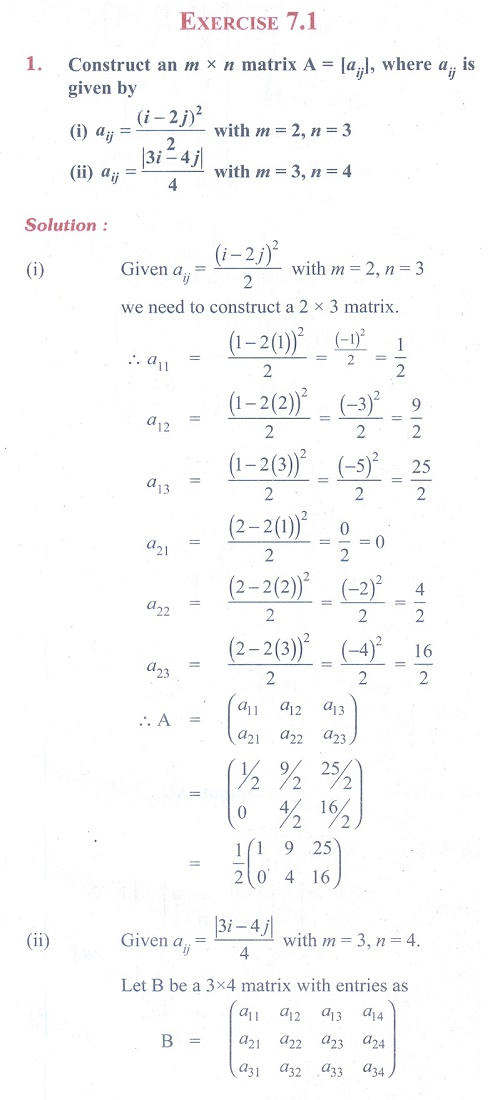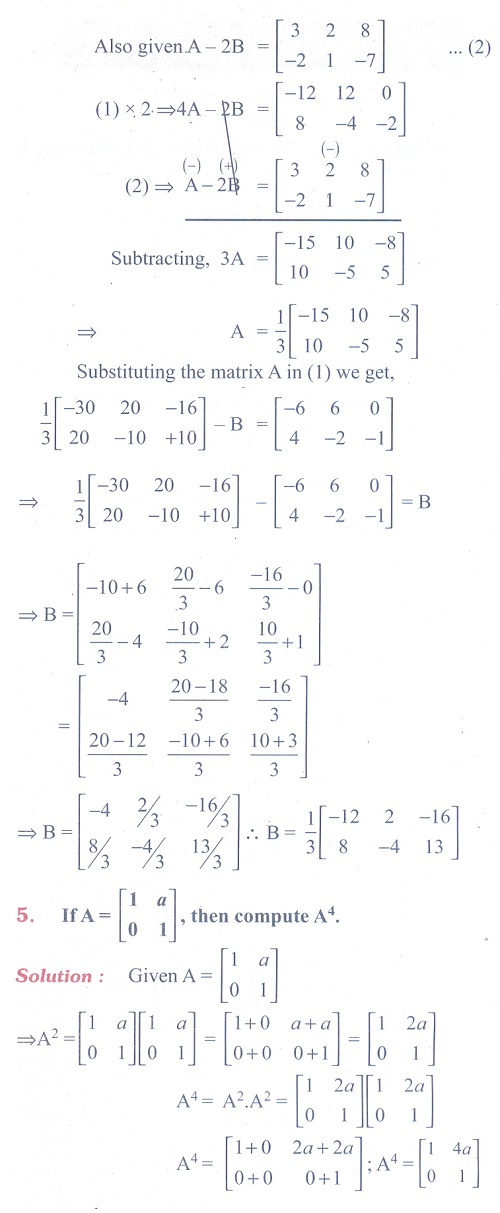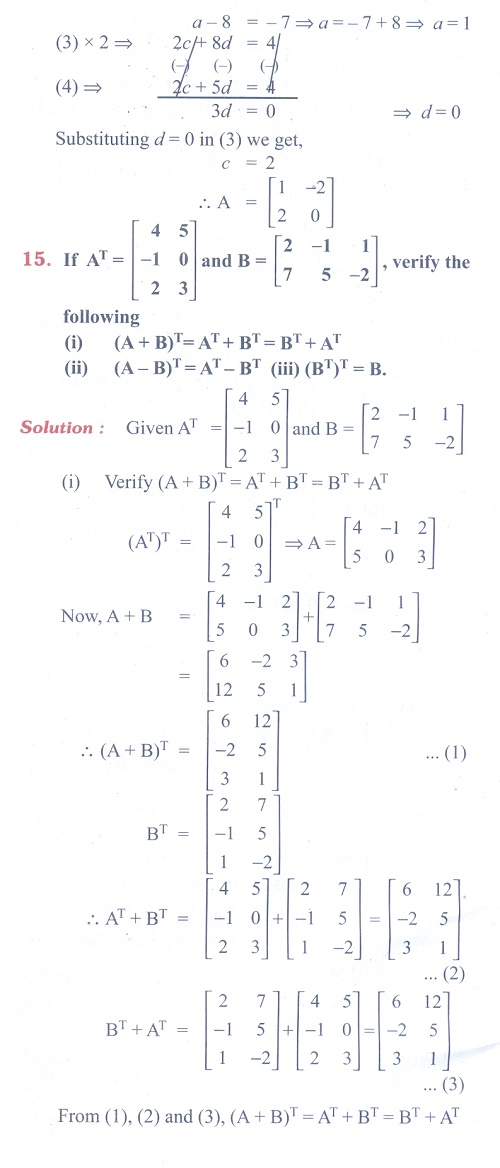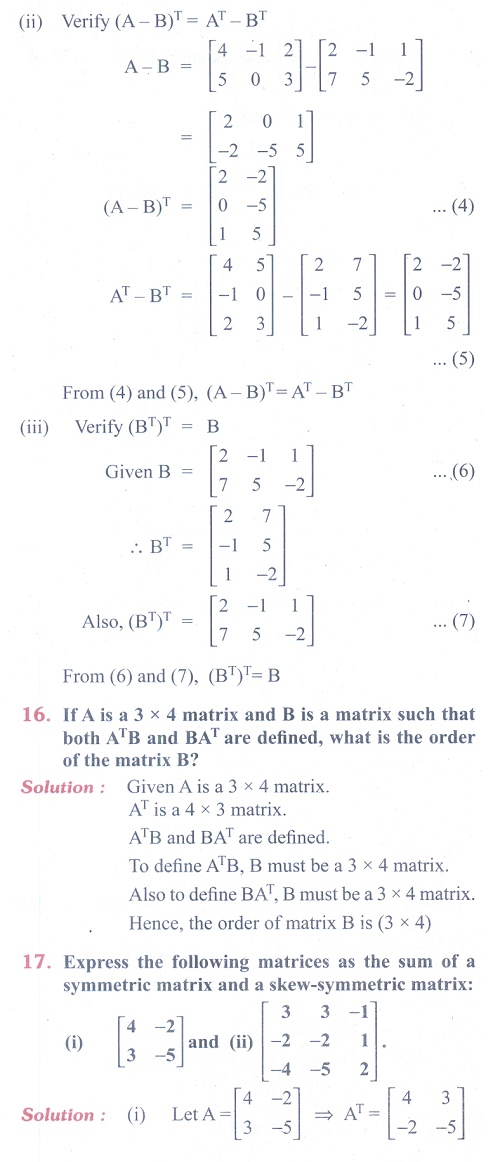(21) Construct the matrix A = [aij ]3×3 , where aij = i − j . State whether A is symmetric or skew-symmetric.

(22) Let A and B be two symmetric matrices. Prove that AB = BA if and only if AB is a symmetric matrix.

(23) If A and B are symmetric matrices of same order, prove that

(i) AB + BA is a symmetric matrix.

(ii) AB - BA is a skew-symmetric matrix.

(24) A shopkeeper in a Nuts and Spices shop makes gift packs of cashew nuts, raisins and almonds.

Pack I contains 100 gm of cashew nuts, 100 gm of raisins and 50 gm of almonds.

Pack-II contains 200 gm of cashew nuts, 100 gm of raisins and 100 gm of almonds.

Pack-III contains 250 gm of cashew nuts, 250 gm of raisins and 150 gm of almonds.

The cost of 50 gm of cashew nuts is Rs.  50, 50 gm of raisins is Rs. 10, and 50 gm of almonds is Rs. 60. What is the cost of each gift pack?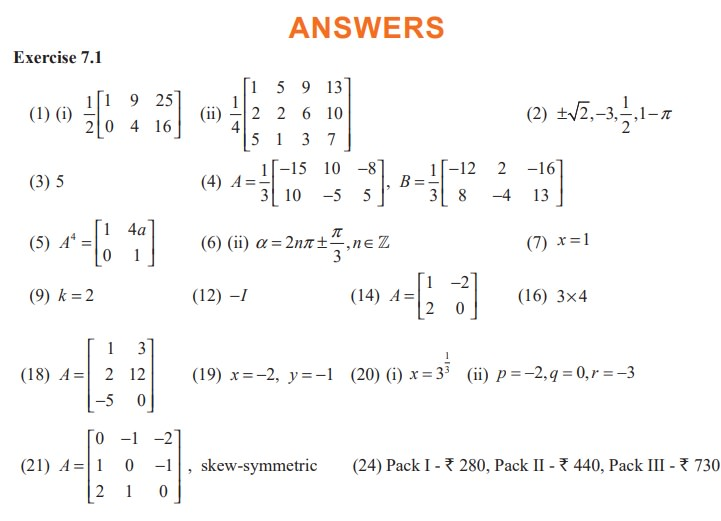Tags : Problem Questions with Answer, Solution | Mathematics , 11th Mathematics : UNIT 7 : Matrices and Determinants
Study Material, Lecturing Notes, Assignment, Reference, Wiki description explanation, brief detail
11th Mathematics : UNIT 7 : Matrices and Determinants : Exercise 7.1: Matrices | Problem Questions with Answer, Solution | Mathematics